Definitions of Square Dance Calls and Concepts

Index -->  Plus  |  A1  |  A2  |  C1  |  C2  |  C3A  |  C3B  |  C4  |  NOL  |
Definitions (Text Only) -->  Plus  |  A1  |  A2  |  C1  |  C2  |  C3A  |  C3B  |  C4  |  NOL  |
 Find call:

 \$B8@8l(B view (admin)

\$BM?\$(\$i\$l\$?%3!<%k\$r8~\$-\$rJQ\$(\$:\$K9T\$\$\$^\$9!%=*\$o\$j\$N0LCV\$O%3!<%k\$rIaDL\$K9T\$C\$?\$H\$-\$HF1\$8\$G\$9\$,(B, \$B8~\$-\$O%3!<%k\$r;O\$a\$k\$H\$-\$HJQ\$o\$j\$^\$;\$s!%(B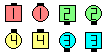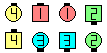Stable In Roll Circulate\$B\$NA0(B \$B8e(B

\$BCmStable \$B\$G\$O\$G\$-\$J\$\$\$H;W\$C\$?\$H\$-(B, \$B8~\$\$\$F\$\$\$kJI\$r3P\$(\$F\$*\$\$\$F%3!<%k\$r9T\$\$(B, \$B3P\$(\$F\$*\$\$\$?JI\$X8~\$-\$rJQ\$(\$k\$H\$\$\$&(B cheat \$B\$,;H\$(\$^\$9!%(B

\$B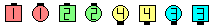\$B\$B;O\$a\$N(B formation: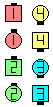Stable As Couples Bend The Line\$B\$N8e(B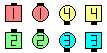As Couples Stable Bend The Line\$B\$N8e(B

1/4 Stable CONCEPT [C4] (Vic Ceder 1983): 90°\$B2s\$k\$^\$G\$OM?\$(\$i\$l\$?%3!<%k\$rIaDL\$K9T\$\$(B, \$B\$=\$N8e(B, \$B%3!<%k\$N;D\$j\$r(B Stable \$B\$G9T\$\$\$^\$9!%(B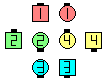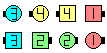1/4 Stable Little More\$B\$NA0(B \$B8e(B

n/4 Stable CONCEPT [C4] (Vic Ceder 1983): \$BN_@QE*\$K(B n · 90°\$B2s\$k\$^\$G\$OM?\$(\$i\$l\$?%3!<%k\$rIaDL\$K9T\$\$(B, \$B\$=\$N8e(B, \$B%3!<%k\$N;D\$j\$r(B Stable \$B\$G9T\$\$\$^\$9!%2sE>\$,N_@QE*\$H\$\$\$&\$3\$H\$O(B, \$BNc\$(\$P(B 90°\$B1&\$X2s\$j\$=\$7\$F(B 90°\$B:8\$X2s\$k\$H\$\$\$&\$3\$H\$O(B, \$B9g7W\$G(B 180°(0°\$B\$G\$J\$/(B) \$B2s\$k\$3\$H\$K\$J\$j\$^\$9!%(B3/4 Stable Exchange The Diamond \$B\$H(B Stable 3/4 Exchange The Diamond \$B\$N0c\$\$\$rNI\$/9M\$(\$F\$_\$F\$/\$@\$5\$\$!%(BCALLERLAB definition for Stable ConceptChoreography for Stable ConceptComments? Questions? Suggestions?

https://www.ceder.net/def/stable.php?level=master&language=japan
23-April-2021 02:38:03
Copyright © 2021 Vic Ceder.  All Rights Reserved.